# Neural machine translation with attention

Stay organized with collections Save and categorize content based on your preferences.

This tutorial demonstrates how to train a sequence-to-sequence (seq2seq) model for Spanish-to-English translation based on the research paper titled Effective Approaches to Attention-based Neural Machine Translation (Luong et al., 2015). This is an advanced example that assumes some knowledge of:

While this architecture is somewhat outdated, it is still a very useful project to work through to get a deeper understanding of sequence-to-sequence models and attention mechanisms (before going on to Transformers).

After training the model in this notebook, you will be able to input a Spanish sentence, such as "¿todavia estan en casa?", and return the English translation: "are you still at home?"

The resulting model is exportable as a tf.saved_model, so it can be used in other TensorFlow environments.

The translation quality is reasonable for a toy example, but the generated attention plot is perhaps more interesting. This shows which parts of the input sentence has the model's attention while translating: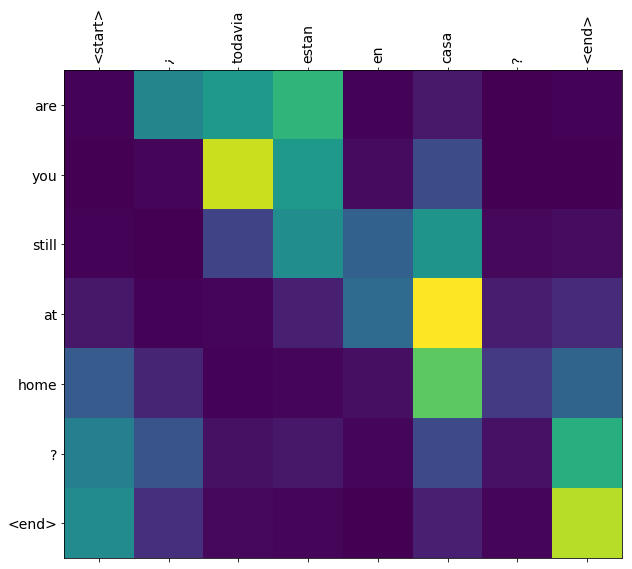## Setup

pip install "tensorflow-text==2.8.*"

import numpy as np

import typing
from typing import Any, Tuple

import tensorflow as tf

import tensorflow_text as tf_text

import matplotlib.pyplot as plt
import matplotlib.ticker as ticker


This tutorial uses a lot of low level API's where it's easy to get shapes wrong. This class is used to check shapes throughout the tutorial.

## The data

The tutorial uses a language dataset provided by Anki. This dataset contains language translation pairs in the format:

May I borrow this book? ¿Puedo tomar prestado este libro?


They have a variety of languages available, but this example uses the English-Spanish dataset.

For convenience, a copy of this dataset is hosted on Google Cloud, but you can also download your own copy. After downloading the dataset, here are the steps you need to take to prepare the data:

1. Add a start and end token to each sentence.
2. Clean the sentences by removing special characters.
3. Create a word index and reverse word index (dictionaries mapping from word → id and id → word).
4. Pad each sentence to a maximum length.
# Download the file
import pathlib

path_to_zip = tf.keras.utils.get_file(
extract=True)

path_to_file = pathlib.Path(path_to_zip).parent/'spa-eng/spa.txt'

Downloading data from http://storage.googleapis.com/download.tensorflow.org/data/spa-eng.zip
2646016/2638744 [==============================] - 0s 0us/step
2654208/2638744 [==============================] - 0s 0us/step

def load_data(path):

lines = text.splitlines()
pairs = [line.split('\t') for line in lines]

inp = [inp for targ, inp in pairs]
targ = [targ for targ, inp in pairs]

return targ, inp

targ, inp = load_data(path_to_file)
print(inp[-1])

Si quieres sonar como un hablante nativo, debes estar dispuesto a practicar diciendo la misma frase una y otra vez de la misma manera en que un músico de banjo practica el mismo fraseo una y otra vez hasta que lo puedan tocar correctamente y en el tiempo esperado.

print(targ[-1])

If you want to sound like a native speaker, you must be willing to practice saying the same sentence over and over in the same way that banjo players practice the same phrase over and over until they can play it correctly and at the desired tempo.


### Create a tf.data dataset

From these arrays of strings you can create a tf.data.Dataset of strings that shuffles and batches them efficiently:

BUFFER_SIZE = len(inp)
BATCH_SIZE = 64

dataset = tf.data.Dataset.from_tensor_slices((inp, targ)).shuffle(BUFFER_SIZE)
dataset = dataset.batch(BATCH_SIZE)

for example_input_batch, example_target_batch in dataset.take(1):
print(example_input_batch[:5])
print()
print(example_target_batch[:5])
break

tf.Tensor(
[b'Tom y Mary se ayudaron mutuamente con los deberes.'
b'Acordamos ese plan.' b'No puedo volver a hacerlo.'
b'\xc3\x89l quiere un iPad 4.' b'Yo pago la cena.'], shape=(5,), dtype=string)

tf.Tensor(
[b'Tom and Mary helped each other with their homework.'
b'We agreed to the plan.' b"I can't do it again." b'He wants an iPad 4.'
b"Dinner's on me."], shape=(5,), dtype=string)


### Text preprocessing

One of the goals of this tutorial is to build a model that can be exported as a tf.saved_model. To make that exported model useful it should take tf.string inputs, and return tf.string outputs: All the text processing happens inside the model.

#### Standardization

The model is dealing with multilingual text with a limited vocabulary. So it will be important to standardize the input text.

The first step is Unicode normalization to split accented characters and replace compatibility characters with their ASCII equivalents.

The tensorflow_text package contains a unicode normalize operation:

example_text = tf.constant('¿Todavía está en casa?')

print(example_text.numpy())
print(tf_text.normalize_utf8(example_text, 'NFKD').numpy())

b'\xc2\xbfTodav\xc3\xada est\xc3\xa1 en casa?'
b'\xc2\xbfTodavi\xcc\x81a esta\xcc\x81 en casa?'


Unicode normalization will be the first step in the text standardization function:

def tf_lower_and_split_punct(text):
# Split accented characters.
text = tf_text.normalize_utf8(text, 'NFKD')
text = tf.strings.lower(text)
# Keep space, a to z, and select punctuation.
text = tf.strings.regex_replace(text, '[^ a-z.?!,¿]', '')
# Add spaces around punctuation.
text = tf.strings.regex_replace(text, '[.?!,¿]', r' \0 ')
# Strip whitespace.
text = tf.strings.strip(text)

text = tf.strings.join(['[START]', text, '[END]'], separator=' ')
return text

print(example_text.numpy().decode())
print(tf_lower_and_split_punct(example_text).numpy().decode())

¿Todavía está en casa?
[START] ¿ todavia esta en casa ? [END]


#### Text Vectorization

This standardization function will be wrapped up in a tf.keras.layers.TextVectorization layer which will handle the vocabulary extraction and conversion of input text to sequences of tokens.

max_vocab_size = 5000

input_text_processor = tf.keras.layers.TextVectorization(
standardize=tf_lower_and_split_punct,
max_tokens=max_vocab_size)


The TextVectorization layer and many other Keras preprocessing layers have an adapt method. This method reads one epoch of the training data, and works a lot like Model.fit. This adapt method initializes the layer based on the data. Here it determines the vocabulary:

input_text_processor.adapt(inp)

# Here are the first 10 words from the vocabulary:
input_text_processor.get_vocabulary()[:10]

['', '[UNK]', '[START]', '[END]', '.', 'que', 'de', 'el', 'a', 'no']


That's the Spanish TextVectorization layer, now build and .adapt() the English one:

output_text_processor = tf.keras.layers.TextVectorization(
standardize=tf_lower_and_split_punct,
max_tokens=max_vocab_size)

output_text_processor.get_vocabulary()[:10]

['', '[UNK]', '[START]', '[END]', '.', 'the', 'i', 'to', 'you', 'tom']


Now these layers can convert a batch of strings into a batch of token IDs:

example_tokens = input_text_processor(example_input_batch)
example_tokens[:3, :10]

<tf.Tensor: shape=(3, 10), dtype=int64, numpy=
array([[   2,   10,   33,   32,   17,    1, 2977,   27,   26,  746],
[   2,    1,   97,  425,    4,    3,    0,    0,    0,    0],
[   2,    9,   55,  358,    8,  198,    4,    3,    0,    0]])>


The get_vocabulary method can be used to convert token IDs back to text:

input_vocab = np.array(input_text_processor.get_vocabulary())
tokens = input_vocab[example_tokens.numpy()]
' '.join(tokens)

'[START] tom y mary se [UNK] mutuamente con los deberes . [END]            '


The returned token IDs are zero-padded. This can easily be turned into a mask:

plt.subplot(1, 2, 1)
plt.pcolormesh(example_tokens)
plt.title('Token IDs')

plt.subplot(1, 2, 2)
plt.pcolormesh(example_tokens != 0)

Text(0.5, 1.0, 'Mask')## The encoder/decoder model

The following diagram shows an overview of the model. At each time-step the decoder's output is combined with a weighted sum over the encoded input, to predict the next word. The diagram and formulas are from Luong's paper.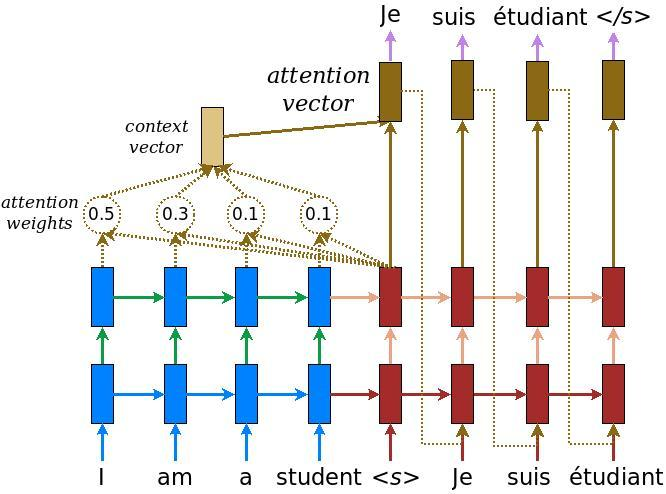Before getting into it define a few constants for the model:

embedding_dim = 256
units = 1024


### The encoder

Start by building the encoder, the blue part of the diagram above.

The encoder:

1. Takes a list of token IDs (from input_text_processor).
2. Looks up an embedding vector for each token (Using a layers.Embedding).
3. Processes the embeddings into a new sequence (Using a layers.GRU).
4. Returns:
• The processed sequence. This will be passed to the attention head.
• The internal state. This will be used to initialize the decoder
class Encoder(tf.keras.layers.Layer):
def __init__(self, input_vocab_size, embedding_dim, enc_units):
super(Encoder, self).__init__()
self.enc_units = enc_units
self.input_vocab_size = input_vocab_size

# The embedding layer converts tokens to vectors
self.embedding = tf.keras.layers.Embedding(self.input_vocab_size,
embedding_dim)

# The GRU RNN layer processes those vectors sequentially.
self.gru = tf.keras.layers.GRU(self.enc_units,
# Return the sequence and state
return_sequences=True,
return_state=True,
recurrent_initializer='glorot_uniform')

def call(self, tokens, state=None):
shape_checker = ShapeChecker()
shape_checker(tokens, ('batch', 's'))

# 2. The embedding layer looks up the embedding for each token.
vectors = self.embedding(tokens)
shape_checker(vectors, ('batch', 's', 'embed_dim'))

# 3. The GRU processes the embedding sequence.
#    output shape: (batch, s, enc_units)
#    state shape: (batch, enc_units)
output, state = self.gru(vectors, initial_state=state)
shape_checker(output, ('batch', 's', 'enc_units'))
shape_checker(state, ('batch', 'enc_units'))

# 4. Returns the new sequence and its state.
return output, state


Here is how it fits together so far:

# Convert the input text to tokens.
example_tokens = input_text_processor(example_input_batch)

# Encode the input sequence.
encoder = Encoder(input_text_processor.vocabulary_size(),
embedding_dim, units)
example_enc_output, example_enc_state = encoder(example_tokens)

print(f'Input batch, shape (batch): {example_input_batch.shape}')
print(f'Input batch tokens, shape (batch, s): {example_tokens.shape}')
print(f'Encoder output, shape (batch, s, units): {example_enc_output.shape}')
print(f'Encoder state, shape (batch, units): {example_enc_state.shape}')

Input batch, shape (batch): (64,)
Input batch tokens, shape (batch, s): (64, 24)
Encoder output, shape (batch, s, units): (64, 24, 1024)
Encoder state, shape (batch, units): (64, 1024)


The encoder returns its internal state so that its state can be used to initialize the decoder.

It's also common for an RNN to return its state so that it can process a sequence over multiple calls. You'll see more of that building the decoder.

### The attention head

The decoder uses attention to selectively focus on parts of the input sequence. The attention takes a sequence of vectors as input for each example and returns an "attention" vector for each example. This attention layer is similar to a layers.GlobalAveragePoling1D but the attention layer performs a weighted average.

Let's look at how this works: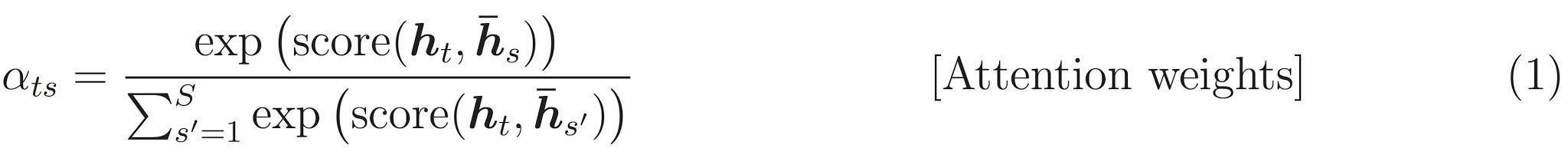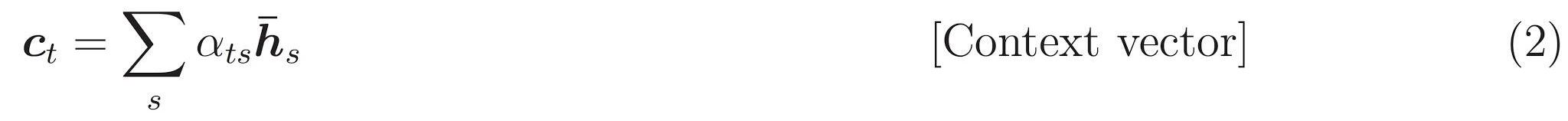Where:

• $$s$$ is the encoder index.
• $$t$$ is the decoder index.
• $$\alpha_{ts}$$ is the attention weights.
• $$h_s$$ is the sequence of encoder outputs being attended to (the attention "key" and "value" in transformer terminology).
• $$h_t$$ is the the decoder state attending to the sequence (the attention "query" in transformer terminology).
• $$c_t$$ is the resulting context vector.
• $$a_t$$ is the final output combining the "context" and "query".

The equations:

1. Calculates the attention weights, $$\alpha_{ts}$$, as a softmax across the encoder's output sequence.
2. Calculates the context vector as the weighted sum of the encoder outputs.

Last is the $$score$$ function. Its job is to calculate a scalar logit-score for each key-query pair. There are two common approaches: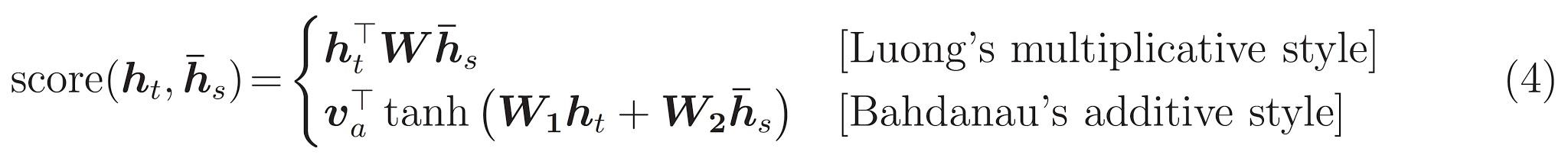This tutorial uses Bahdanau's additive attention. TensorFlow includes implementations of both as layers.Attention and layers.AdditiveAttention. The class below handles the weight matrices in a pair of layers.Dense layers, and calls the built-in implementation.

class BahdanauAttention(tf.keras.layers.Layer):
def __init__(self, units):
super().__init__()
# For Eqn. (4), the  Bahdanau attention
self.W1 = tf.keras.layers.Dense(units, use_bias=False)
self.W2 = tf.keras.layers.Dense(units, use_bias=False)

def call(self, query, value, mask):
shape_checker = ShapeChecker()
shape_checker(query, ('batch', 't', 'query_units'))
shape_checker(value, ('batch', 's', 'value_units'))

# From Eqn. (4), W1@ht.
w1_query = self.W1(query)
shape_checker(w1_query, ('batch', 't', 'attn_units'))

# From Eqn. (4), W2@hs.
w2_key = self.W2(value)
shape_checker(w2_key, ('batch', 's', 'attn_units'))

query_mask = tf.ones(tf.shape(query)[:-1], dtype=bool)

context_vector, attention_weights = self.attention(
inputs = [w1_query, value, w2_key],
return_attention_scores = True,
)
shape_checker(context_vector, ('batch', 't', 'value_units'))
shape_checker(attention_weights, ('batch', 't', 's'))

return context_vector, attention_weights


### Test the Attention layer

Create a BahdanauAttention layer:

attention_layer = BahdanauAttention(units)


This layer takes 3 inputs:

• The query: This will be generated by the decoder, later.
• The value: This Will be the output of the encoder.
• The mask: To exclude the padding, example_tokens != 0
(example_tokens != 0).shape

TensorShape([64, 24])


The vectorized implementation of the attention layer lets you pass a batch of sequences of query vectors and a batch of sequence of value vectors. The result is:

1. A batch of sequences of result vectors the size of the queries.
2. A batch of attention maps with size (query_length, value_length).
# Later, the decoder will generate this attention query
example_attention_query = tf.random.normal(shape=[len(example_tokens), 2, 10])

# Attend to the encoded tokens
context_vector, attention_weights = attention_layer(
query=example_attention_query,
value=example_enc_output,

print(f'Attention result shape: (batch_size, query_seq_length, units):           {context_vector.shape}')
print(f'Attention weights shape: (batch_size, query_seq_length, value_seq_length): {attention_weights.shape}')

Attention result shape: (batch_size, query_seq_length, units):           (64, 2, 1024)
Attention weights shape: (batch_size, query_seq_length, value_seq_length): (64, 2, 24)


The attention weights should sum to 1.0 for each sequence.

Here are the attention weights across the sequences at t=0:

plt.subplot(1, 2, 1)
plt.pcolormesh(attention_weights[:, 0, :])
plt.title('Attention weights')

plt.subplot(1, 2, 2)
plt.pcolormesh(example_tokens != 0)

Text(0.5, 1.0, 'Mask')Because of the small-random initialization the attention weights are all close to 1/(sequence_length). If you zoom in on the weights for a single sequence, you can see that there is some small variation that the model can learn to expand, and exploit.

attention_weights.shape

TensorShape([64, 2, 24])

attention_slice = attention_weights[0, 0].numpy()
attention_slice = attention_slice[attention_slice != 0]


[<matplotlib.lines.Line2D at 0x7f233d8b4490>]
<Figure size 432x288 with 0 Axes>### The decoder

The decoder's job is to generate predictions for the next output token.

1. The decoder receives the complete encoder output.
2. It uses an RNN to keep track of what it has generated so far.
3. It uses its RNN output as the query to the attention over the encoder's output, producing the context vector.
4. It combines the RNN output and the context vector using Equation 3 (below) to generate the "attention vector".
5. It generates logit predictions for the next token based on the "attention vector".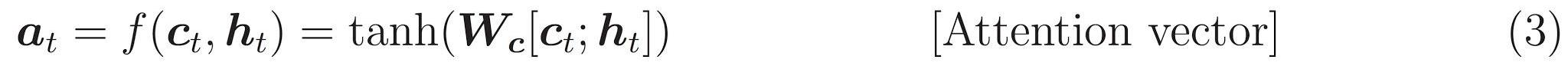Here is the Decoder class and its initializer. The initializer creates all the necessary layers.

class Decoder(tf.keras.layers.Layer):
def __init__(self, output_vocab_size, embedding_dim, dec_units):
super(Decoder, self).__init__()
self.dec_units = dec_units
self.output_vocab_size = output_vocab_size
self.embedding_dim = embedding_dim

# For Step 1. The embedding layer converts token IDs to vectors
self.embedding = tf.keras.layers.Embedding(self.output_vocab_size,
embedding_dim)

# For Step 2. The RNN keeps track of what's been generated so far.
self.gru = tf.keras.layers.GRU(self.dec_units,
return_sequences=True,
return_state=True,
recurrent_initializer='glorot_uniform')

# For step 3. The RNN output will be the query for the attention layer.
self.attention = BahdanauAttention(self.dec_units)

# For step 4. Eqn. (3): converting ct to at
self.Wc = tf.keras.layers.Dense(dec_units, activation=tf.math.tanh,
use_bias=False)

# For step 5. This fully connected layer produces the logits for each
# output token.
self.fc = tf.keras.layers.Dense(self.output_vocab_size)


The call method for this layer takes and returns multiple tensors. Organize those into simple container classes:

class DecoderInput(typing.NamedTuple):
new_tokens: Any
enc_output: Any

class DecoderOutput(typing.NamedTuple):
logits: Any
attention_weights: Any


Here is the implementation of the call method:

def call(self,
inputs: DecoderInput,
state=None) -> Tuple[DecoderOutput, tf.Tensor]:
shape_checker = ShapeChecker()
shape_checker(inputs.new_tokens, ('batch', 't'))
shape_checker(inputs.enc_output, ('batch', 's', 'enc_units'))

if state is not None:
shape_checker(state, ('batch', 'dec_units'))

# Step 1. Lookup the embeddings
vectors = self.embedding(inputs.new_tokens)
shape_checker(vectors, ('batch', 't', 'embedding_dim'))

# Step 2. Process one step with the RNN
rnn_output, state = self.gru(vectors, initial_state=state)

shape_checker(rnn_output, ('batch', 't', 'dec_units'))
shape_checker(state, ('batch', 'dec_units'))

# Step 3. Use the RNN output as the query for the attention over the
# encoder output.
context_vector, attention_weights = self.attention(
shape_checker(context_vector, ('batch', 't', 'dec_units'))
shape_checker(attention_weights, ('batch', 't', 's'))

# Step 4. Eqn. (3): Join the context_vector and rnn_output
#     [ct; ht] shape: (batch t, value_units + query_units)
context_and_rnn_output = tf.concat([context_vector, rnn_output], axis=-1)

# Step 4. Eqn. (3): at = tanh(Wc@[ct; ht])
attention_vector = self.Wc(context_and_rnn_output)
shape_checker(attention_vector, ('batch', 't', 'dec_units'))

# Step 5. Generate logit predictions:
logits = self.fc(attention_vector)
shape_checker(logits, ('batch', 't', 'output_vocab_size'))

return DecoderOutput(logits, attention_weights), state

Decoder.call = call


The encoder processes its full input sequence with a single call to its RNN. This implementation of the decoder can do that as well for efficient training. But this tutorial will run the decoder in a loop for a few reasons:

• Flexibility: Writing the loop gives you direct control over the training procedure.
• Clarity: It's possible to do masking tricks and use layers.RNN, or tfa.seq2seq APIs to pack this all into a single call. But writing it out as a loop may be clearer.

Now try using this decoder.

decoder = Decoder(output_text_processor.vocabulary_size(),
embedding_dim, units)


The decoder takes 4 inputs.

• new_tokens - The last token generated. Initialize the decoder with the "[START]" token.
• enc_output - Generated by the Encoder.
• mask - A boolean tensor indicating where tokens != 0
• state - The previous state output from the decoder (the internal state of the decoder's RNN). Pass None to zero-initialize it. The original paper initializes it from the encoder's final RNN state.
# Convert the target sequence, and collect the "[START]" tokens
example_output_tokens = output_text_processor(example_target_batch)

start_index = output_text_processor.get_vocabulary().index('[START]')
first_token = tf.constant([[start_index]] * example_output_tokens.shape)

# Run the decoder
dec_result, dec_state = decoder(
inputs = DecoderInput(new_tokens=first_token,
enc_output=example_enc_output,
state = example_enc_state
)

print(f'logits shape: (batch_size, t, output_vocab_size) {dec_result.logits.shape}')
print(f'state shape: (batch_size, dec_units) {dec_state.shape}')

logits shape: (batch_size, t, output_vocab_size) (64, 1, 5000)
state shape: (batch_size, dec_units) (64, 1024)


Sample a token according to the logits:

sampled_token = tf.random.categorical(dec_result.logits[:, 0, :], num_samples=1)


Decode the token as the first word of the output:

vocab = np.array(output_text_processor.get_vocabulary())
first_word = vocab[sampled_token.numpy()]
first_word[:5]

array([['letters'],
['attracted'],
['crime'],
['this']], dtype='<U16')


Now use the decoder to generate a second set of logits.

• Pass the same enc_output and mask, these haven't changed.
• Pass the sampled token as new_tokens.
• Pass the decoder_state the decoder returned last time, so the RNN continues with a memory of where it left off last time.
dec_result, dec_state = decoder(
DecoderInput(sampled_token,
example_enc_output,
state=dec_state)

sampled_token = tf.random.categorical(dec_result.logits[:, 0, :], num_samples=1)
first_word = vocab[sampled_token.numpy()]
first_word[:5]

array([['suspicious'],
['bald'],
['motivated'],
['definitely'],
['reported']], dtype='<U16')


## Training

Now that you have all the model components, it's time to start training the model. You'll need:

• A loss function and optimizer to perform the optimization.
• A training step function defining how to update the model for each input/target batch.
• A training loop to drive the training and save checkpoints.

### Define the loss function

class MaskedLoss(tf.keras.losses.Loss):
def __init__(self):
self.loss = tf.keras.losses.SparseCategoricalCrossentropy(
from_logits=True, reduction='none')

def __call__(self, y_true, y_pred):
shape_checker = ShapeChecker()
shape_checker(y_true, ('batch', 't'))
shape_checker(y_pred, ('batch', 't', 'logits'))

# Calculate the loss for each item in the batch.
loss = self.loss(y_true, y_pred)
shape_checker(loss, ('batch', 't'))

# Mask off the losses on padding.
mask = tf.cast(y_true != 0, tf.float32)

# Return the total.
return tf.reduce_sum(loss)


### Implement the training step

Start by subclassing tf.keras.Model, where the training process will be implemented as the train_step method on this TrainTranslator subclass of tf.keras.Model. Refer to Customize what happens in Model.fit for details.

Here, the train_step method is a wrapper around the _train_step implementation which will come later. This wrapper includes a switch to turn on and off tf.function compilation to make debugging easier.

class TrainTranslator(tf.keras.Model):
def __init__(self, embedding_dim, units,
input_text_processor,
output_text_processor,
use_tf_function=True):
super().__init__()
# Build the encoder and decoder
encoder = Encoder(input_text_processor.vocabulary_size(),
embedding_dim, units)
decoder = Decoder(output_text_processor.vocabulary_size(),
embedding_dim, units)

self.encoder = encoder
self.decoder = decoder
self.input_text_processor = input_text_processor
self.output_text_processor = output_text_processor
self.use_tf_function = use_tf_function
self.shape_checker = ShapeChecker()

def train_step(self, inputs):
self.shape_checker = ShapeChecker()
if self.use_tf_function:
return self._tf_train_step(inputs)
else:
return self._train_step(inputs)


Overall the implementation for the Model.train_step method is as follows:

1. Receive a batch of input_text, target_text from the tf.data.Dataset.
2. Convert those raw text inputs to token-embeddings and masks.
3. Run the encoder on the input_tokens to get the encoder_output and encoder_state.
4. Initialize the decoder state and loss.
5. Loop over the target_tokens:
1. Run the decoder one step at a time.
2. Calculate the loss for each step.
3. Accumulate the average loss.
6. Calculate the gradient of the loss and use the optimizer to apply updates to the model's trainable_variables.

The _preprocess method, added below, implements steps #1 and #2:

def _preprocess(self, input_text, target_text):
self.shape_checker(input_text, ('batch',))
self.shape_checker(target_text, ('batch',))

# Convert the text to token IDs
input_tokens = self.input_text_processor(input_text)
target_tokens = self.output_text_processor(target_text)
self.shape_checker(input_tokens, ('batch', 's'))
self.shape_checker(target_tokens, ('batch', 't'))

# Convert IDs to masks.
input_mask = input_tokens != 0

target_mask = target_tokens != 0


TrainTranslator._preprocess = _preprocess


The _train_step method, added below, handles the remaining steps except for actually running the decoder:

def _train_step(self, inputs):
input_text, target_text = inputs

target_tokens, target_mask) = self._preprocess(input_text, target_text)

max_target_length = tf.shape(target_tokens)

with tf.GradientTape() as tape:
# Encode the input
enc_output, enc_state = self.encoder(input_tokens)
self.shape_checker(enc_output, ('batch', 's', 'enc_units'))
self.shape_checker(enc_state, ('batch', 'enc_units'))

# Initialize the decoder's state to the encoder's final state.
# This only works if the encoder and decoder have the same number of
# units.
dec_state = enc_state
loss = tf.constant(0.0)

for t in tf.range(max_target_length-1):
# Pass in two tokens from the target sequence:
# 1. The current input to the decoder.
# 2. The target for the decoder's next prediction.
new_tokens = target_tokens[:, t:t+2]
step_loss, dec_state = self._loop_step(new_tokens, input_mask,
enc_output, dec_state)
loss = loss + step_loss

# Average the loss over all non padding tokens.
average_loss = loss / tf.reduce_sum(tf.cast(target_mask, tf.float32))

# Apply an optimization step
variables = self.trainable_variables

# Return a dict mapping metric names to current value
return {'batch_loss': average_loss}

TrainTranslator._train_step = _train_step


The _loop_step method, added below, executes the decoder and calculates the incremental loss and new decoder state (dec_state).

def _loop_step(self, new_tokens, input_mask, enc_output, dec_state):
input_token, target_token = new_tokens[:, 0:1], new_tokens[:, 1:2]

# Run the decoder one step.
decoder_input = DecoderInput(new_tokens=input_token,
enc_output=enc_output,

dec_result, dec_state = self.decoder(decoder_input, state=dec_state)
self.shape_checker(dec_result.logits, ('batch', 't1', 'logits'))
self.shape_checker(dec_result.attention_weights, ('batch', 't1', 's'))
self.shape_checker(dec_state, ('batch', 'dec_units'))

# self.loss returns the total for non-padded tokens
y = target_token
y_pred = dec_result.logits
step_loss = self.loss(y, y_pred)

return step_loss, dec_state

TrainTranslator._loop_step = _loop_step


### Test the training step

Build a TrainTranslator, and configure it for training using the Model.compile method:

translator = TrainTranslator(
embedding_dim, units,
input_text_processor=input_text_processor,
output_text_processor=output_text_processor,
use_tf_function=False)

# Configure the loss and optimizer
translator.compile(
)


Test out the train_step. For a text model like this the loss should start near:

np.log(output_text_processor.vocabulary_size())

8.517193191416238

%%time
for n in range(10):
print(translator.train_step([example_input_batch, example_target_batch]))
print()

{'batch_loss': <tf.Tensor: shape=(), dtype=float32, numpy=7.6307936>}
{'batch_loss': <tf.Tensor: shape=(), dtype=float32, numpy=7.598738>}
{'batch_loss': <tf.Tensor: shape=(), dtype=float32, numpy=7.5392323>}
{'batch_loss': <tf.Tensor: shape=(), dtype=float32, numpy=7.3694654>}
{'batch_loss': <tf.Tensor: shape=(), dtype=float32, numpy=6.728599>}
{'batch_loss': <tf.Tensor: shape=(), dtype=float32, numpy=5.274465>}
{'batch_loss': <tf.Tensor: shape=(), dtype=float32, numpy=4.77829>}
{'batch_loss': <tf.Tensor: shape=(), dtype=float32, numpy=4.6239724>}
{'batch_loss': <tf.Tensor: shape=(), dtype=float32, numpy=4.3668847>}
{'batch_loss': <tf.Tensor: shape=(), dtype=float32, numpy=4.1383567>}

CPU times: user 6.55 s, sys: 0 ns, total: 6.55 s
Wall time: 6.52 s


While it's easier to debug without a tf.function it does give a performance boost. So now that the _train_step method is working, try the tf.function-wrapped _tf_train_step, to maximize performance while training:

@tf.function(input_signature=[[tf.TensorSpec(dtype=tf.string, shape=[None]),
tf.TensorSpec(dtype=tf.string, shape=[None])]])
def _tf_train_step(self, inputs):
return self._train_step(inputs)

TrainTranslator._tf_train_step = _tf_train_step

translator.use_tf_function = True


The first call will be slow, because it traces the function.

translator.train_step([example_input_batch, example_target_batch])

2022-08-04 12:15:48.641343: E tensorflow/core/grappler/optimizers/meta_optimizer.cc:828] function_optimizer failed: INVALID_ARGUMENT: Input 6 of node gradient_tape/while/while_grad/body/_531/gradient_tape/while/gradients/while/decoder_1/gru_3/PartitionedCall_grad/PartitionedCall was passed variant from gradient_tape/while/while_grad/body/_531/gradient_tape/while/gradients/while/decoder_1/gru_3/PartitionedCall_grad/TensorListPopBack_2:1 incompatible with expected float.
2022-08-04 12:15:48.745874: E tensorflow/core/grappler/optimizers/meta_optimizer.cc:828] layout failed: OUT_OF_RANGE: src_output = 25, but num_outputs is only 25
when importing GraphDef to MLIR module in GrapplerHook
2022-08-04 12:15:48.996369: W tensorflow/core/common_runtime/process_function_library_runtime.cc:932] Ignoring multi-device function optimization failure: INVALID_ARGUMENT: Input 1 of node while/body/_1/while/TensorListPushBack_56 was passed float from while/body/_1/while/decoder_1/gru_3/PartitionedCall:6 incompatible with expected variant.
{'batch_loss': <tf.Tensor: shape=(), dtype=float32, numpy=4.009605>}


But after that it's usually 2-3x faster than the eager train_step method:

%%time
for n in range(10):
print(translator.train_step([example_input_batch, example_target_batch]))
print()

{'batch_loss': <tf.Tensor: shape=(), dtype=float32, numpy=4.0036325>}
{'batch_loss': <tf.Tensor: shape=(), dtype=float32, numpy=4.01246>}
{'batch_loss': <tf.Tensor: shape=(), dtype=float32, numpy=3.9528773>}
{'batch_loss': <tf.Tensor: shape=(), dtype=float32, numpy=3.8428462>}
{'batch_loss': <tf.Tensor: shape=(), dtype=float32, numpy=3.7700226>}
{'batch_loss': <tf.Tensor: shape=(), dtype=float32, numpy=3.7392578>}
{'batch_loss': <tf.Tensor: shape=(), dtype=float32, numpy=3.6912892>}
{'batch_loss': <tf.Tensor: shape=(), dtype=float32, numpy=3.645986>}
{'batch_loss': <tf.Tensor: shape=(), dtype=float32, numpy=3.589823>}
{'batch_loss': <tf.Tensor: shape=(), dtype=float32, numpy=3.5579007>}

CPU times: user 4.61 s, sys: 809 ms, total: 5.42 s
Wall time: 1.93 s


A good test of a new model is to see that it can overfit a single batch of input. Try it, the loss should quickly go to zero:

losses = []
for n in range(100):
print('.', end='')
logs = translator.train_step([example_input_batch, example_target_batch])
losses.append(logs['batch_loss'].numpy())

print()
plt.plot(losses)

....................................................................................................Now that you're confident that the training step is working, build a fresh copy of the model to train from scratch:

train_translator = TrainTranslator(
embedding_dim, units,
input_text_processor=input_text_processor,
output_text_processor=output_text_processor)

# Configure the loss and optimizer
train_translator.compile(
)


### Train the model

While there's nothing wrong with writing your own custom training loop, implementing the Model.train_step method, as in the previous section, allows you to run Model.fit and avoid rewriting all that boiler-plate code.

This tutorial only trains for a couple of epochs, so use a callbacks.Callback to collect the history of batch losses, for plotting:

class BatchLogs(tf.keras.callbacks.Callback):
def __init__(self, key):
self.key = key
self.logs = []

def on_train_batch_end(self, n, logs):
self.logs.append(logs[self.key])

batch_loss = BatchLogs('batch_loss')

train_translator.fit(dataset, epochs=3,
callbacks=[batch_loss])

Epoch 1/3
2022-08-04 12:16:14.863105: E tensorflow/core/grappler/optimizers/meta_optimizer.cc:828] layout failed: OUT_OF_RANGE: src_output = 25, but num_outputs is only 25
when importing GraphDef to MLIR module in GrapplerHook
2022-08-04 12:16:15.129140: W tensorflow/core/common_runtime/process_function_library_runtime.cc:932] Ignoring multi-device function optimization failure: INVALID_ARGUMENT: Input 1 of node StatefulPartitionedCall/while/body/_59/while/TensorListPushBack_56 was passed float from StatefulPartitionedCall/while/body/_59/while/decoder_2/gru_5/PartitionedCall:6 incompatible with expected variant.
1859/1859 [==============================] - 305s 161ms/step - batch_loss: 2.0345
Epoch 2/3
1859/1859 [==============================] - 301s 162ms/step - batch_loss: 1.0443
Epoch 3/3
1859/1859 [==============================] - 300s 162ms/step - batch_loss: 0.8145
<keras.callbacks.History at 0x7f22c467f220>

plt.plot(batch_loss.logs)
plt.ylim([0, 3])
plt.xlabel('Batch #')
plt.ylabel('CE/token')

Text(0, 0.5, 'CE/token')The visible jumps in the plot are at the epoch boundaries.

## Translate

Now that the model is trained, implement a function to execute the full text => text translation.

For this the model needs to invert the text => token IDs mapping provided by the output_text_processor. It also needs to know the IDs for special tokens. This is all implemented in the constructor for the new class. The implementation of the actual translate method will follow.

Overall this is similar to the training loop, except that the input to the decoder at each time step is a sample from the decoder's last prediction.

class Translator(tf.Module):

def __init__(self, encoder, decoder, input_text_processor,
output_text_processor):
self.encoder = encoder
self.decoder = decoder
self.input_text_processor = input_text_processor
self.output_text_processor = output_text_processor

self.output_token_string_from_index = (
tf.keras.layers.StringLookup(
vocabulary=output_text_processor.get_vocabulary(),
invert=True))

# The output should never generate padding, unknown, or start.
index_from_string = tf.keras.layers.StringLookup(
token_mask_ids = index_from_string(['', '[UNK]', '[START]']).numpy()

token_mask = np.zeros([index_from_string.vocabulary_size()], dtype=np.bool)

self.start_token = index_from_string(tf.constant('[START]'))
self.end_token = index_from_string(tf.constant('[END]'))

translator = Translator(
encoder=train_translator.encoder,
decoder=train_translator.decoder,
input_text_processor=input_text_processor,
output_text_processor=output_text_processor,
)

/tmpfs/tmp/ipykernel_44101/100404259.py:21: DeprecationWarning: np.bool is a deprecated alias for the builtin bool. To silence this warning, use bool by itself. Doing this will not modify any behavior and is safe. If you specifically wanted the numpy scalar type, use np.bool_ here.
Deprecated in NumPy 1.20; for more details and guidance: https://numpy.org/devdocs/release/1.20.0-notes.html#deprecations
token_mask = np.zeros([index_from_string.vocabulary_size()], dtype=np.bool)


### Convert token IDs to text

The first method to implement is tokens_to_text which converts from token IDs to human readable text.

def tokens_to_text(self, result_tokens):
shape_checker = ShapeChecker()
shape_checker(result_tokens, ('batch', 't'))
result_text_tokens = self.output_token_string_from_index(result_tokens)
shape_checker(result_text_tokens, ('batch', 't'))

result_text = tf.strings.reduce_join(result_text_tokens,
axis=1, separator=' ')
shape_checker(result_text, ('batch'))

result_text = tf.strings.strip(result_text)
shape_checker(result_text, ('batch',))
return result_text

Translator.tokens_to_text = tokens_to_text


Input some random token IDs and see what it generates:

example_output_tokens = tf.random.uniform(
shape=[5, 2], minval=0, dtype=tf.int64,
maxval=output_text_processor.vocabulary_size())
translator.tokens_to_text(example_output_tokens).numpy()

array([b'walking solving', b'blames superior', b'spending duck',
b'piano sip', b'background friday'], dtype=object)


### Sample from the decoder's predictions

This function takes the decoder's logit outputs and samples token IDs from that distribution:

def sample(self, logits, temperature):
shape_checker = ShapeChecker()
# 't' is usually 1 here.
shape_checker(logits, ('batch', 't', 'vocab'))

# Set the logits for all masked tokens to -inf, so they are never chosen.
logits = tf.where(self.token_mask, -np.inf, logits)

if temperature == 0.0:
new_tokens = tf.argmax(logits, axis=-1)
else:
logits = tf.squeeze(logits, axis=1)
new_tokens = tf.random.categorical(logits/temperature,
num_samples=1)

shape_checker(new_tokens, ('batch', 't'))

return new_tokens

Translator.sample = sample


Test run this function on some random inputs:

example_logits = tf.random.normal([5, 1, output_text_processor.vocabulary_size()])
example_output_tokens = translator.sample(example_logits, temperature=1.0)
example_output_tokens

<tf.Tensor: shape=(5, 1), dtype=int64, numpy=
array([,
,
[ 652],
[  76],
])>


### Implement the translation loop

Here is a complete implementation of the text to text translation loop.

This implementation collects the results into python lists, before using tf.concat to join them into tensors.

This implementation statically unrolls the graph out to max_length iterations. This is okay with eager execution in python.

def translate_unrolled(self,
input_text, *,
max_length=50,
return_attention=True,
temperature=1.0):
batch_size = tf.shape(input_text)
input_tokens = self.input_text_processor(input_text)
enc_output, enc_state = self.encoder(input_tokens)

dec_state = enc_state
new_tokens = tf.fill([batch_size, 1], self.start_token)

result_tokens = []
attention = []
done = tf.zeros([batch_size, 1], dtype=tf.bool)

for _ in range(max_length):
dec_input = DecoderInput(new_tokens=new_tokens,
enc_output=enc_output,

dec_result, dec_state = self.decoder(dec_input, state=dec_state)

attention.append(dec_result.attention_weights)

new_tokens = self.sample(dec_result.logits, temperature)

# If a sequence produces an end_token, set it done
done = done | (new_tokens == self.end_token)
# Once a sequence is done it only produces 0-padding.
new_tokens = tf.where(done, tf.constant(0, dtype=tf.int64), new_tokens)

# Collect the generated tokens
result_tokens.append(new_tokens)

if tf.executing_eagerly() and tf.reduce_all(done):
break

# Convert the list of generates token ids to a list of strings.
result_tokens = tf.concat(result_tokens, axis=-1)
result_text = self.tokens_to_text(result_tokens)

if return_attention:
attention_stack = tf.concat(attention, axis=1)
return {'text': result_text, 'attention': attention_stack}
else:
return {'text': result_text}

Translator.translate = translate_unrolled


Run it on a simple input:

%%time
input_text = tf.constant([
'hace mucho frio aqui.', # "It's really cold here."
'Esta es mi vida.', # "This is my life.""
])

result = translator.translate(
input_text = input_text)

print(result['text'].numpy().decode())
print(result['text'].numpy().decode())
print()

its very cold here .
this is my life .

CPU times: user 148 ms, sys: 0 ns, total: 148 ms
Wall time: 144 ms


If you want to export this model you'll need to wrap this method in a tf.function. This basic implementation has a few issues if you try to do that:

1. The resulting graphs are very large and take a few seconds to build, save or load.
2. You can't break from a statically unrolled loop, so it will always run max_length iterations, even if all the outputs are done. But even then it's marginally faster than eager execution.
@tf.function(input_signature=[tf.TensorSpec(dtype=tf.string, shape=[None])])
def tf_translate(self, input_text):
return self.translate(input_text)

Translator.tf_translate = tf_translate


Run the tf.function once to compile it:

%%time
result = translator.tf_translate(
input_text = input_text)

CPU times: user 18.9 s, sys: 0 ns, total: 18.9 s
Wall time: 18.8 s

%%time
result = translator.tf_translate(
input_text = input_text)

print(result['text'].numpy().decode())
print(result['text'].numpy().decode())
print()

its cold here .
this is my life .

CPU times: user 119 ms, sys: 0 ns, total: 119 ms
Wall time: 76.6 ms


### [Optional] Use a symbolic loop

Translator.translate = translate_symbolic


The initial implementation used python lists to collect the outputs. This uses tf.range as the loop iterator, allowing tf.autograph to convert the loop. The biggest change in this implementation is the use of tf.TensorArray instead of python list to accumulate tensors. tf.TensorArray is required to collect a variable number of tensors in graph mode.

With eager execution this implementation performs on par with the original:

%%time
result = translator.translate(
input_text = input_text)

print(result['text'].numpy().decode())
print(result['text'].numpy().decode())
print()

it is very cold here .
this is my life .

CPU times: user 191 ms, sys: 0 ns, total: 191 ms
Wall time: 186 ms


But when you wrap it in a tf.function you'll notice two differences.

@tf.function(input_signature=[tf.TensorSpec(dtype=tf.string, shape=[None])])
def tf_translate(self, input_text):
return self.translate(input_text)

Translator.tf_translate = tf_translate


First: Graph creation is much faster (~10x), since it doesn't create max_iterations copies of the model.

%%time
result = translator.tf_translate(
input_text = input_text)

CPU times: user 1.07 s, sys: 0 ns, total: 1.07 s
Wall time: 1.05 s


Second: The compiled function is much faster on small inputs (5x on this example), because it can break out of the loop.

%%time
result = translator.tf_translate(
input_text = input_text)

print(result['text'].numpy().decode())
print(result['text'].numpy().decode())
print()

its very cold here .
this is my life .

CPU times: user 34.2 ms, sys: 0 ns, total: 34.2 ms
Wall time: 14 ms


### Visualize the process

The attention weights returned by the translate method show where the model was "looking" when it generated each output token.

So the sum of the attention over the input should return all ones:

a = result['attention']

print(np.sum(a, axis=-1))

[1.0000001  1.         0.99999994 1.         1.         1.0000001 ]


Here is the attention distribution for the first output step of the first example. Note how the attention is now much more focused than it was for the untrained model:

_ = plt.bar(range(len(a[0, :])), a[0, :])Since there is some rough alignment between the input and output words, you expect the attention to be focused near the diagonal:

plt.imshow(np.array(a), vmin=0.0)

<matplotlib.image.AxesImage at 0x7f21d0287970>Here is some code to make a better attention plot:

### Labeled attention plots

i=0
plot_attention(result['attention'][i], input_text[i], result['text'][i])

/tmpfs/tmp/ipykernel_44101/143215515.py:14: UserWarning: FixedFormatter should only be used together with FixedLocator
ax.set_xticklabels([''] + sentence, fontdict=fontdict, rotation=90)
/tmpfs/tmp/ipykernel_44101/143215515.py:15: UserWarning: FixedFormatter should only be used together with FixedLocator
ax.set_yticklabels([''] + predicted_sentence, fontdict=fontdict)Translate a few more sentences and plot them:

%%time
three_input_text = tf.constant([
# This is my life.
'Esta es mi vida.',
# Are they still home?
'¿Todavía están en casa?',
# Try to find out.'
'Tratar de descubrir.',
])

result = translator.tf_translate(three_input_text)

for tr in result['text']:
print(tr.numpy().decode())

print()

this is my life .
are you still at home ?
lets try to figure out .

CPU times: user 39.7 ms, sys: 34 ms, total: 73.6 ms
Wall time: 17 ms

result['text']

<tf.Tensor: shape=(3,), dtype=string, numpy=
array([b'this is my life .', b'are you still at home ?',
b'lets try to figure out .'], dtype=object)>

i = 0
plot_attention(result['attention'][i], three_input_text[i], result['text'][i])

/tmpfs/tmp/ipykernel_44101/143215515.py:14: UserWarning: FixedFormatter should only be used together with FixedLocator
ax.set_xticklabels([''] + sentence, fontdict=fontdict, rotation=90)
/tmpfs/tmp/ipykernel_44101/143215515.py:15: UserWarning: FixedFormatter should only be used together with FixedLocator
ax.set_yticklabels([''] + predicted_sentence, fontdict=fontdict)i = 1
plot_attention(result['attention'][i], three_input_text[i], result['text'][i])

/tmpfs/tmp/ipykernel_44101/143215515.py:14: UserWarning: FixedFormatter should only be used together with FixedLocator
ax.set_xticklabels([''] + sentence, fontdict=fontdict, rotation=90)
/tmpfs/tmp/ipykernel_44101/143215515.py:15: UserWarning: FixedFormatter should only be used together with FixedLocator
ax.set_yticklabels([''] + predicted_sentence, fontdict=fontdict)i = 2
plot_attention(result['attention'][i], three_input_text[i], result['text'][i])

/tmpfs/tmp/ipykernel_44101/143215515.py:14: UserWarning: FixedFormatter should only be used together with FixedLocator
ax.set_xticklabels([''] + sentence, fontdict=fontdict, rotation=90)
/tmpfs/tmp/ipykernel_44101/143215515.py:15: UserWarning: FixedFormatter should only be used together with FixedLocator
ax.set_yticklabels([''] + predicted_sentence, fontdict=fontdict)The short sentences often work well, but if the input is too long the model literally loses focus and stops providing reasonable predictions. There are two main reasons for this:

1. The model was trained with teacher-forcing feeding the correct token at each step, regardless of the model's predictions. The model could be made more robust if it were sometimes fed its own predictions.
2. The model only has access to its previous output through the RNN state. If the RNN state gets corrupted, there's no way for the model to recover. Transformers solve this by using self-attention in the encoder and decoder.
long_input_text = tf.constant([inp[-1]])

import textwrap
print('Expected output:\n', '\n'.join(textwrap.wrap(targ[-1])))

Expected output:
If you want to sound like a native speaker, you must be willing to
practice saying the same sentence over and over in the same way that
banjo players practice the same phrase over and over until they can
play it correctly and at the desired tempo.

result = translator.tf_translate(long_input_text)

i = 0
plot_attention(result['attention'][i], long_input_text[i], result['text'][i])
_ = plt.suptitle('This never works')

/tmpfs/tmp/ipykernel_44101/143215515.py:14: UserWarning: FixedFormatter should only be used together with FixedLocator
ax.set_xticklabels([''] + sentence, fontdict=fontdict, rotation=90)
/tmpfs/tmp/ipykernel_44101/143215515.py:15: UserWarning: FixedFormatter should only be used together with FixedLocator
ax.set_yticklabels([''] + predicted_sentence, fontdict=fontdict)## Export

Once you have a model you're satisfied with you might want to export it as a tf.saved_model for use outside of this python program that created it.

Since the model is a subclass of tf.Module (through keras.Model), and all the functionality for export is compiled in a tf.function the model should export cleanly with tf.saved_model.save:

Now that the function has been traced it can be exported using saved_model.save:

tf.saved_model.save(translator, 'translator',
signatures={'serving_default': translator.tf_translate})

2022-08-04 12:31:40.935911: W tensorflow/python/util/util.cc:368] Sets are not currently considered sequences, but this may change in the future, so consider avoiding using them.
WARNING:absl:Found untraced functions such as encoder_2_layer_call_fn, encoder_2_layer_call_and_return_conditional_losses, decoder_2_layer_call_fn, decoder_2_layer_call_and_return_conditional_losses, embedding_4_layer_call_fn while saving (showing 5 of 24). These functions will not be directly callable after loading.
INFO:tensorflow:Assets written to: translator/assets
INFO:tensorflow:Assets written to: translator/assets

reloaded = tf.saved_model.load('translator')

%%time

for tr in result['text']:
print(tr.numpy().decode())

print()

this is my life .
are you still at home ?
try to find out .

CPU times: user 31.6 ms, sys: 7.17 ms, total: 38.7 ms
Wall time: 16.1 ms


## Next steps

[{ "type": "thumb-down", "id": "missingTheInformationINeed", "label":"Missing the information I need" },{ "type": "thumb-down", "id": "tooComplicatedTooManySteps", "label":"Too complicated / too many steps" },{ "type": "thumb-down", "id": "outOfDate", "label":"Out of date" },{ "type": "thumb-down", "id": "samplesCodeIssue", "label":"Samples / code issue" },{ "type": "thumb-down", "id": "otherDown", "label":"Other" }]
[{ "type": "thumb-up", "id": "easyToUnderstand", "label":"Easy to understand" },{ "type": "thumb-up", "id": "solvedMyProblem", "label":"Solved my problem" },{ "type": "thumb-up", "id": "otherUp", "label":"Other" }]